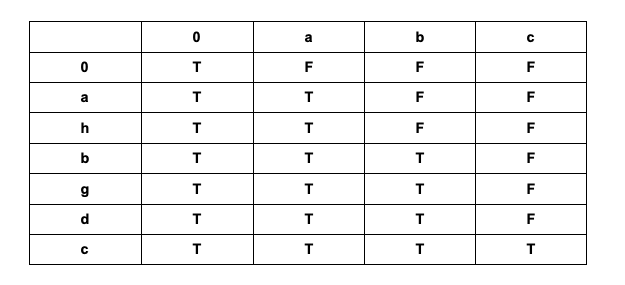### LeetCode• ㊗️
• 大家
• offer
• 多多！

## Problem

Given two strings s and t, check if s is a subsequence of t.

A subsequence of a string is a new string that is formed from the original string by deleting some (can be none) of the characters without disturbing the relative positions of the remaining characters. (i.e., “ace” is a subsequence of “abcde” while “aec” is not).

Example 1:

Input: s = "abc", t = "ahbgdc"
Output: true


Example 2:

Input: s = "axc", t = "ahbgdc"
Output: false


## Code

class Solution {
public boolean isSubsequence(String s, String t) {
if(s == null || s.length() == 0) return true;

int curr = 0;
for(char c : t.toCharArray()) {
if(curr == s.length()) return true;
if(c == s.charAt(curr)) {
curr++;
}
}

return curr == s.length();
}
}


DP 解法class Solution {
public boolean isSubsequence(String s, String t) {
int sLen = s.length();
int tLen = t.length();

boolean[][] dp = new boolean[tLen + 1][sLen + 1];
for(int i = 0; i <= tLen; i++){
dp[i] = true;
}

for(int i = 1; i <= tLen; i++){
for(int j = 1; j <= sLen; j++){
if(dp[i - 1][j] == true){
dp[i][j] = true;
} else if(t.charAt(i - 1) == s.charAt(j - 1) && dp[i - 1][j - 1] == true){
dp[i][j] = true;
}
}
}

return dp[tLen][sLen];
}
}